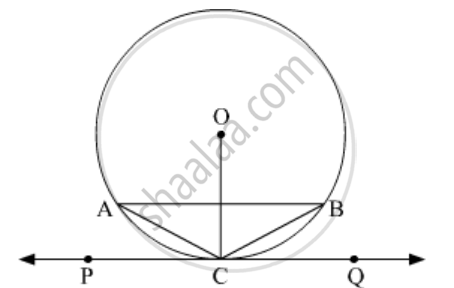# Prove that the Tangent Drawn at the Mid-point of an Arc of a Circle is Parallel to the Chord Joining the End Points of the Arc. - Mathematics

Diagram
Sum

Prove that the tangent drawn at the mid-point of an arc of a circle is parallel to the chord joining the end points of the arc.

#### Solution 1In the given figure, C is the mid point of the minor arc AB of the circle with centre O.
PQ is the tangent to the given circle through point C.
To Prove: Tangent drawn at the mid point of the arc

$\stackrel\frown{AB}$ of a circle is parallel to the chord joining the end point of the arc $\stackrel\frown{AB}$

i.e AB || PQ.
Proof: C is the mid point of the minor arc AB
⇒ minor arc AC = minor arc BC
⇒ AC = BC
Thus, ∆ABC is an iscosceles triangle.
Therefore, the perpendicular bisector of side AB of ∆ABC passes through the vertex C.
We know that the perpendicular bisector of a chord passes through the centre of the circle.
Since AB is a chord of the circle so, the perpendicular bisector of AB passes through the centre O.
Thus, it is clear that the perpendicular bisector of AB passes through the points O and C.
Therefore,

$AB \perp OC$

Now, PQ is the tangent to the circle through the point C on the circle.
Therefore,

$PQ \perp OC \left[ \text{Tangent to a circle is perpendicular to its radius through the point of contact} \right]$

#### Solution 2Let us draw a circle in which AMB is an arc and M is the mid-point of the arc AMB.

Joined AM and MB. Also TT' is a tangent at point M on the circle.

To Prove: AB || TT'

Proof: As M is the mid point of Arc AMB

Arc AM = Arc MB

AM = MB  .......[As equal chords cuts equal arcs]

∠ABM = ∠BAM  .......[Angles opposite to equal sides are equal] 

Now, ∠BMT' = ∠BAM  ......[Angle between tangent and the chord equals angle made by the chord in alternate segment] 

From  and 

∠ABM = ∠BMT'

So, AB || TT' [two lines are parallel if the interior alternate angles are equal]

Hence Proved!

Concept: Tangent to a Circle
Is there an error in this question or solution?

#### APPEARS IN

NCERT Mathematics Exemplar Class 10
Chapter 9 Circles
Exercise 9.4 | Q 9 | Page 111
RD Sharma Class 10 Maths
Chapter 8 Circles
Exercise 8.2 | Q 16 | Page 35
Share